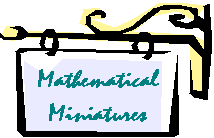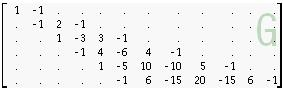# Gottfried Helms

https://www.helms-net.de

D - Kassel - Dez 2006

https://go.helms-net.de## Numbertheoretical matricesIn this section I'm presenting a collection of basic numbertheoretical matrices, for instance the Pascalmatrix (binomialmatrix), matrices of Bernoulli-/Euler- and other polynomials, Stirling-numbers and some interesting and systematic relations between them. (The matrices are lower-triangular number-arrays of infinite dimension.)

This collection is primarily a compilation of that matrices and their basic properties. Then their relations are presented and discussed. The occuring identities are in most cases well known, but especially the eigensystem-decompositions (and their consequences) seem to be unique in this collection.

Most of the relations were foundheuristically by playing around with that matrices in the style of experimenting with a toolbox. The proofs for the given identities and relations shall be added as I have time (and as far as I'm able to derive them). When I was looking in literature (mostly internet resources) I found most of these identities spreaded over various resources: here I try to put them together in a more systematic context.

An unexpected but interesting extension popped up very soon: the problems of divergent summation: matrix-operations with that (in most cases) integer-numberarrays means to assign values to divergent summation in a very natural way.

A references section is far from being complete. I'll add the references while I'm extending the already present chapters as well while I'm creating the missing ones.

Gottfried Helms 07'2010             (first version: 12'2006)## Intro and notation

The basic definitions and conventions, the short names of the basic matrices.

Intro and notations

List of matrices

## 1 Basic matrices and properties

Pascal-/Binomialmatrix (09'2007)

A generalization of the subdiagonal/matrix-exponential-approach leads to versions of the Laguerre-matrix and other, yet anonymous matrices.

The signed Pascal-matrix and their eigensystems (not yet ready; see older version Pascalmatrix so far)Gp-matrices (Bernoulli~ & Euler-polynomials)

The fundamental matrices of Bernoulli/Euler-polynomials BY/EY, the (Jacob Bernoullian) matrices G (Gp & Gm) and the (Eulerian) matrix Ep (not yet ready; Gp is described so far in older version Pascalmatrix)

Stirlingnumbers (02'2007): the matrices St1 and St2 of Stirlingnumbers of 1'st and 2'nd kind, Bell-numbers.

Gaussmatrix(11'2007)

The "Gaussian"-matrix occured to me when I tried to find derivatives and integrals of the gaussian function. The matrix is then the triangle of coefficients of z which are needed to express the derivatives. It occured, that even this matrix is closely related to the binomialmatrix by the same subdiagonal/matrix-exponential-scheme as a very nice and simple generalization.Eulermatrix (08'2009)

The Eulermatrix / matrix of Eulerian numbers occurs among others in the problem of summing-of-like powers. However, it has interesting features:

·        its rowsums are related to the gamma and the alternating zeta-function,

·        we can use fractional row-indexes and that relation still holds.

After that I propose a procedure for the summation of a strongly divergent series: for

su  = 0! – 1! + 2! – 3! + 4! – … + …

I arrive at the same famous value 0.59634736… (Gompertz-constant) as we get it if we use L.Euler's first solution involving integrals or Borel-summation or the EI()-function.

This is the key for the more general computation of

su (x) = 0! + 1! x + 2! x2 + 3! x3 + 4! x4 + … + … at x=-1

A discussion in the usenet-discussionlist sci.math can be found here: discuss1ZV seems more and more the core matrix of all that considerations. This is not surprising: it is vertically read the matrix of zeta-sequences and horizontally read of the geometric series: the most basic aspects that may be studied in number-theories. (12'2007)## 2 Special aspects of some matrices

Generalized Bernoulli Recursion (06'2008)

I found a sort of "generalized bernoulli recursion". This is a very special recursion different from the ususal recursive definition of bernoulli-numbers. The derivation of this recursion also exhibits interesting relations between the most basic sequences of numbers like Bernoulli-numbers, Eta-numbers, reciprocals of powers of 2, reciprocals of binomial-coefficients and more.

After an online search, this recursive definition came out to be already known, but the sources didn't mention this type of derivation and the direct visible connection to other sequences.

The definition also gives some formulae for surprising identities for weighted sums of bernoulli-numbers and the other sets of numbers.## 4 Sums and divergent summation using matrices

Summation: Introductory remarks about divergent summation: Cesaro/Euler-summation in matrix-representation; A direct summation-approach for some special applications

(not yet ready; see older version Summation so far)

An approach to the problem of, as it was solved by Jacob Bernoulli, when he introduced his polynomial coefficients, which are now named after him.

Here I discuss a derivation simply by using the geometric series of the Pascalmatrix, which leads to a summing-operator for alternating sums of like powers and then also to non-alternating sums. The derivation here involves the basic definition of the values of the eta- and the zeta-function, thus leads from eta/zeta-values to the eta/bernoulli-numbers as a special case.Bell-numbers and divergent summation with Bell-numbers: (08'2008)

I look at the introduction of the Bell-numbers by E.T. Bell and reconsider the recursive definition in terms of my matrix-notation. I proceed to find a way to sum the Bell-numbers of all orders with a method of divergent summation.## 10 various, partly "esoteric" findings, discussions and loose ends

An interesting problem, mentioned by Erdoes, is, whether the sums-of-like-powers from 1m to nm can equal (n+1)m . Using the summation-property of the Gp-matrix (as mentioned for instance in sums-of-like-powers) the problem is translated into a polynomial expression. For these expressions I search for zeros, which, if positive integer were solutions of the Erdoes-problem. The article is an investigation of this problem.

A "reciprocal" variant of the zeta-summation along the idea of H.Hasse. Helmut Hasse in a 1930-article gave a summation-scheme for values of the zeta-function at negative exponents and showed the relation between values of the zeta-function and bernoulli-numbers. His scheme is generalizable for summing to even any zeta-value the same way, which fits nicely in this matrix-context. However, the property, that his sums involve only finite many terms is valid for negative integer arguments of the zeta-function only. This approach is revisited and an experimental variant is discussed, which applies the same method to zeta-values at positive exponents. While I didn't come to a useful result it may be an initialization to evaluate this idea in greater depth.A discussion of the problem of computing the inverse/ reciprocal of the Vandermonde-matrix. Since the Vandermondematrix ZV can be LU-decomposed into a pascalmatrix and a scaled Stirling-2'dn kind-matrix (which was for instance used in a H. Hasse article in 1930) an inverse for finite dimension can be computed by the simple inversion of the triangular components. The difficult composition of the entries of the inverse vandermonde-matrix with changing dimensions is shown as dependent only of the number of rows/columns, which are used from the pascal/St1-matrix, which are constant with changing dimensions. Also an approach using ideas of divergent summation is introduced.

Zeta-sums: a couple of sums-of-bernoulli-numbers, rational sums-of-zeta-values- identitiesPowertowers

I introduce iteration of functions, which are defined by powerseries, with powers of appropriate matrices. In the initial article I use the Vandermonde- and Stirling-matrices to discuss iterated b^x and b^x -1 . I proceed to a heuristic about an infinite series of powertowers of increasing heights. This is just exploration!

A shorter and more focused version is this.

See more articles about iterated exponentiation in the section "tetration" of the parent directory.

## Older versions:

Pascalmatrix : introduces the concept, the Pascalmatrix, Gp-matrix as eigensystem, St2/St1-matrices; can be used until the chapter "Bernoulli-/GP-matrix" is rewritten. (German version)

Special properties of the Pascalmatrix and divergent Summation: more extensive discussion of Pascalmatrix plus a chapter about divergent summation using the binomial and other matrices (still manuscript version, will successively be elaborated (and replaced) by the separate articles above).

Touches the most aspects which are discussed in the newer articles in terms of my first heuristic findings and propositions.G.Helms Vers 21.8.2009